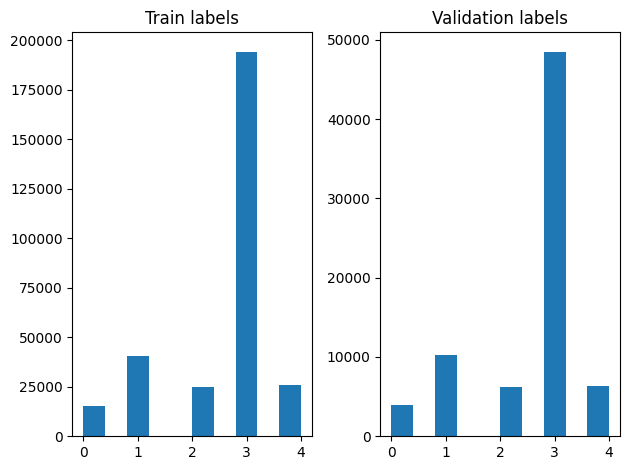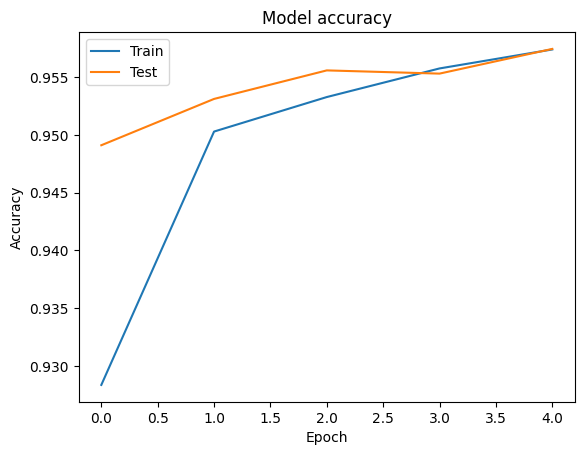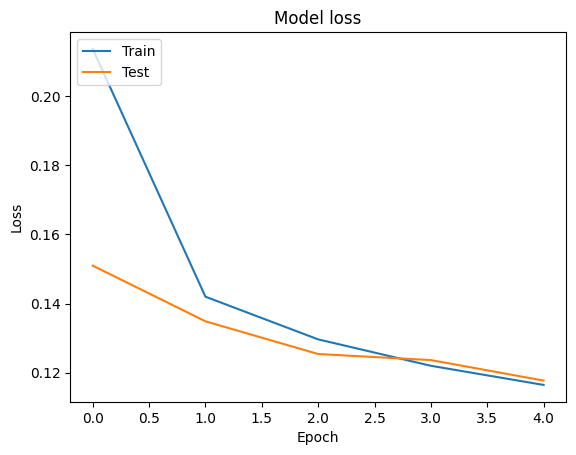# Bangla Article Classification With TF-Hub

This Colab is a demonstration of using Tensorflow Hub for text classification in non-English/local languages. Here we choose Bangla as the local language and use pretrained word embeddings to solve a multiclass classification task where we classify Bangla news articles in 5 categories. The pretrained embeddings for Bangla comes from fastText which is a library by Facebook with released pretrained word vectors for 157 languages.

We'll use TF-Hub's pretrained embedding exporter for converting the word embeddings to a text embedding module first and then use the module to train a classifier with tf.keras, Tensorflow's high level user friendly API to build deep learning models. Even if we are using fastText embeddings here, it's possible to export any other embeddings pretrained from other tasks and quickly get results with Tensorflow hub.

## Setup

````# https://github.com/pypa/setuptools/issues/1694#issuecomment-466010982`
`pip install gdown --no-use-pep517`
```
````sudo apt-get install -y unzip`
```
```Reading package lists...
Building dependency tree...
The following packages were automatically installed and are no longer required:
libatasmart4 libblockdev-fs2 libblockdev-loop2 libblockdev-part-err2
libblockdev-part2 libblockdev-swap2 libblockdev-utils2 libblockdev2
libparted-fs-resize0 libxmlb2
Use 'sudo apt autoremove' to remove them.
0 upgraded, 0 newly installed, 0 to remove and 126 not upgraded.
```
``````import os

import tensorflow as tf
import tensorflow_hub as hub

import gdown
import numpy as np
from sklearn.metrics import classification_report
import matplotlib.pyplot as plt
import seaborn as sns
``````

# Dataset

We will use BARD (Bangla Article Dataset) which has around 376,226 articles collected from different Bangla news portals and labelled with 5 categories: economy, state, international, sports, and entertainment. We download the file from Google Drive this (bit.ly/BARD_DATASET) link is referring to from this GitHub repository.

``````gdown.download(
output='bard.zip',
quiet=True
)
``````
```'bard.zip'
```
````unzip -qo bard.zip`
```

# Export pretrained word vectors to TF-Hub module

TF-Hub provides some useful scripts for converting word embeddings to TF-hub text embedding modules here. To make the module for Bangla or any other languages, we simply have to download the word embedding `.txt` or `.vec` file to the same directory as `export_v2.py` and run the script.

The exporter reads the embedding vectors and exports it to a Tensorflow SavedModel. A SavedModel contains a complete TensorFlow program including weights and graph. TF-Hub can load the SavedModel as a module, which we will use to build the model for text classification. Since we are using `tf.keras` to build the model, we will use hub.KerasLayer, which provides a wrapper for a TF-Hub module to use as a Keras Layer.

First we will get our word embeddings from fastText and embedding exporter from TF-Hub repo.

````curl -O https://dl.fbaipublicfiles.com/fasttext/vectors-crawl/cc.bn.300.vec.gz`
`curl -O https://raw.githubusercontent.com/tensorflow/hub/master/examples/text_embeddings_v2/export_v2.py`
`gunzip -qf cc.bn.300.vec.gz --k`
```
```% Total    % Received % Xferd  Average Speed   Time    Time     Time  Current
100  840M  100  840M    0     0  41.8M      0  0:00:20  0:00:20 --:--:-- 42.3M
% Total    % Received % Xferd  Average Speed   Time    Time     Time  Current
100  7500  100  7500    0     0  50675      0 --:--:-- --:--:-- --:--:-- 50675
```

Then, we will run the exporter script on our embedding file. Since fastText embeddings have a header line and are pretty large (around 3.3 GB for Bangla after converting to a module) we ignore the first line and export only the first 100, 000 tokens to the text embedding module.

````python export_v2.py --embedding_file=cc.bn.300.vec --export_path=text_module --num_lines_to_ignore=1 --num_lines_to_use=100000`
```
```INFO:tensorflow:Assets written to: text_module/assets
I0317 11:10:25.262115 140175947175744 builder_impl.py:797] Assets written to: text_module/assets
```
``````module_path = "text_module"
embedding_layer = hub.KerasLayer(module_path, trainable=False)
``````

The text embedding module takes a batch of sentences in a 1D tensor of strings as input and outputs the embedding vectors of shape (batch_size, embedding_dim) corresponding to the sentences. It preprocesses the input by splitting on spaces. Word embeddings are combined to sentence embeddings with the `sqrtn` combiner(See here). For demonstration we pass a list of Bangla words as input and get the corresponding embedding vectors.

``````embedding_layer(['বাস', 'বসবাস', 'ট্রেন', 'যাত্রী', 'ট্রাক'])
``````
```<tf.Tensor: shape=(5, 300), dtype=float64, numpy=
array([[ 0.0462, -0.0355,  0.0129, ...,  0.0025, -0.0966,  0.0216],
[-0.0631, -0.0051,  0.085 , ...,  0.0249, -0.0149,  0.0203],
[ 0.1371, -0.069 , -0.1176, ...,  0.029 ,  0.0508, -0.026 ],
[ 0.0532, -0.0465, -0.0504, ...,  0.02  , -0.0023,  0.0011],
[ 0.0908, -0.0404, -0.0536, ..., -0.0275,  0.0528,  0.0253]])>
```

# Convert to Tensorflow Dataset

Since the dataset is really large instead of loading the entire dataset in memory we will use a generator to yield samples in run-time in batches using Tensorflow Dataset functions. The dataset is also very imbalanced, so, before using the generator, we will shuffle the dataset.

``````dir_names = ['economy', 'sports', 'entertainment', 'state', 'international']

file_paths = []
labels = []
for i, dir in enumerate(dir_names):
file_names = ["/".join([dir, name]) for name in os.listdir(dir)]
file_paths += file_names
labels += [i] * len(os.listdir(dir))

np.random.seed(42)
permutation = np.random.permutation(len(file_paths))

file_paths = np.array(file_paths)[permutation]
labels = np.array(labels)[permutation]
``````

We can check the distribution of labels in the training and validation examples after shuffling.

``````train_frac = 0.8
train_size = int(len(file_paths) * train_frac)
``````
``````# plot training vs validation distribution
plt.subplot(1, 2, 1)
plt.hist(labels[0:train_size])
plt.title("Train labels")
plt.subplot(1, 2, 2)
plt.hist(labels[train_size:])
plt.title("Validation labels")
plt.tight_layout()
``````To create a Dataset using a generator, we first write a generator function which reads each of the articles from `file_paths` and the labels from the label array, and yields one training example at each step. We pass this generator function to the `tf.data.Dataset.from_generator` method and specify the output types. Each training example is a tuple containing an article of `tf.string` data type and one-hot encoded label. We split the dataset with a train-validation split of 80-20 using `tf.data.Dataset.skip` and `tf.data.Dataset.take` methods.

``````def load_file(path, label):
``````
``````def make_datasets(train_size):
batch_size = 256

train_files = file_paths[:train_size]
train_labels = labels[:train_size]
train_ds = tf.data.Dataset.from_tensor_slices((train_files, train_labels))
train_ds = train_ds.batch(batch_size).prefetch(tf.data.AUTOTUNE)

test_files = file_paths[train_size:]
test_labels = labels[train_size:]
test_ds = tf.data.Dataset.from_tensor_slices((test_files, test_labels))
test_ds = test_ds.batch(batch_size).prefetch(tf.data.AUTOTUNE)

return train_ds, test_ds
``````
``````train_data, validation_data = make_datasets(train_size)
``````

# Model Training and Evaluation

Since we have already added a wrapper around our module to use it as any other layer in Keras, we can create a small Sequential model which is a linear stack of layers. We can add our text embedding module with `model.add` just like any other layer. We compile the model by specifying the loss and optimizer and train it for 10 epochs. The `tf.keras` API can handle Tensorflow Datasets as input, so we can pass a Dataset instance to the fit method for model training. Since we are using the generator function, `tf.data` will handle generating the samples, batching them and feeding them to the model.

## Model

``````def create_model():
model = tf.keras.Sequential([
tf.keras.layers.Input(shape=[], dtype=tf.string),
embedding_layer,
tf.keras.layers.Dense(64, activation="relu"),
tf.keras.layers.Dense(16, activation="relu"),
tf.keras.layers.Dense(5),
])
model.compile(loss=tf.losses.SparseCategoricalCrossentropy(from_logits=True),
return model
``````
``````model = create_model()
# Create earlystopping callback
early_stopping_callback = tf.keras.callbacks.EarlyStopping(monitor='val_loss', min_delta=0, patience=3)
``````

## Training

``````history = model.fit(train_data,
validation_data=validation_data,
epochs=5,
callbacks=[early_stopping_callback])
``````
```Epoch 1/5
1176/1176 [==============================] - 28s 21ms/step - loss: 0.2255 - accuracy: 0.9257 - val_loss: 0.1532 - val_accuracy: 0.9480
Epoch 2/5
1176/1176 [==============================] - 24s 20ms/step - loss: 0.1415 - accuracy: 0.9499 - val_loss: 0.1353 - val_accuracy: 0.9518
Epoch 3/5
1176/1176 [==============================] - 24s 20ms/step - loss: 0.1304 - accuracy: 0.9532 - val_loss: 0.1308 - val_accuracy: 0.9535
Epoch 4/5
1176/1176 [==============================] - 24s 20ms/step - loss: 0.1226 - accuracy: 0.9554 - val_loss: 0.1225 - val_accuracy: 0.9560
Epoch 5/5
1176/1176 [==============================] - 24s 21ms/step - loss: 0.1172 - accuracy: 0.9571 - val_loss: 0.1224 - val_accuracy: 0.9552
```

## Evaluation

We can visualize the accuracy and loss curves for training and validation data using the `tf.keras.callbacks.History` object returned by the `tf.keras.Model.fit` method, which contains the loss and accuracy value for each epoch.

``````# Plot training & validation accuracy values
plt.plot(history.history['accuracy'])
plt.plot(history.history['val_accuracy'])
plt.title('Model accuracy')
plt.ylabel('Accuracy')
plt.xlabel('Epoch')
plt.legend(['Train', 'Test'], loc='upper left')
plt.show()

# Plot training & validation loss values
plt.plot(history.history['loss'])
plt.plot(history.history['val_loss'])
plt.title('Model loss')
plt.ylabel('Loss')
plt.xlabel('Epoch')
plt.legend(['Train', 'Test'], loc='upper left')
plt.show()
``````## Prediction

We can get the predictions for the validation data and check the confusion matrix to see the model's performance for each of the 5 classes. Because `tf.keras.Model.predict` method returns an n-d array for probabilities for each class, they can be converted to class labels using `np.argmax`.

``````y_pred = model.predict(validation_data)
``````
```294/294 [==============================] - 5s 15ms/step
```
``````y_pred = np.argmax(y_pred, axis=1)
``````
``````samples = file_paths[0:3]
for i, sample in enumerate(samples):
f = open(sample)
print(text[0:100])
print("True Class: ", sample.split("/"))
print("Predicted Class: ", dir_names[y_pred[i]])
f.close()
``````
```বিগত কয়েক বছরের ধারাবাহিকতায় এবারও ঢাকা বিশ্ববিদ্যালয়ের চারুকলা অনুষদের বকুলতলায় বর্ষা উৎসবের আয়োজন
True Class:  entertainment
Predicted Class:  state

কুশিয়ারা নদীরক্ষা বাঁধ নির্মাণে গাফিলতির কারণেই সিলেটের ওসমানীনগর উপজেলার ২১টি গ্রাম বন্যার পানিতে
True Class:  state
Predicted Class:  state

বিশ্বকাপে ব্রাজিলই সবচেয়ে সফল দেশ। সর্বোচ্চ পাঁচবার বিশ্বকাপ শিরোপা জিতেছে সেলেসাওরা। কিন্তু নিজ দে
True Class:  sports
Predicted Class:  state
```

## Compare Performance

Now we can take the correct labels for the validation data from `labels` and compare them with our predictions to get a classification_report.

``````y_true = np.array(labels[train_size:])
``````
``````print(classification_report(y_true, y_pred, target_names=dir_names))
``````
```precision    recall  f1-score   support

economy       0.80      0.80      0.80      3897
sports       0.98      0.99      0.99     10204
entertainment       0.93      0.91      0.92      6256
state       0.97      0.98      0.97     48512
international       0.95      0.89      0.92      6377

accuracy                           0.96     75246
macro avg       0.93      0.91      0.92     75246
weighted avg       0.96      0.96      0.96     75246
```

We can also compare our model's performance with the published results obtained in the original paper, which had a 0.96 precision .The original authors described many preprocessing steps performed on the dataset, such as dropping punctuations and digits, removing top 25 most frequest stop words. As we can see in the `classification_report`, we also manage to obtain a 0.96 precision and accuracy after training for only 5 epochs without any preprocessing!

In this example, when we created the Keras layer from our embedding module, we set the parameter`trainable=False`, which means the embedding weights will not be updated during training. Try setting it to `True` to reach around 97% accuracy using this dataset after only 2 epochs.

[{ "type": "thumb-down", "id": "missingTheInformationINeed", "label":"Missing the information I need" },{ "type": "thumb-down", "id": "tooComplicatedTooManySteps", "label":"Too complicated / too many steps" },{ "type": "thumb-down", "id": "outOfDate", "label":"Out of date" },{ "type": "thumb-down", "id": "samplesCodeIssue", "label":"Samples / code issue" },{ "type": "thumb-down", "id": "otherDown", "label":"Other" }]
[{ "type": "thumb-up", "id": "easyToUnderstand", "label":"Easy to understand" },{ "type": "thumb-up", "id": "solvedMyProblem", "label":"Solved my problem" },{ "type": "thumb-up", "id": "otherUp", "label":"Other" }]